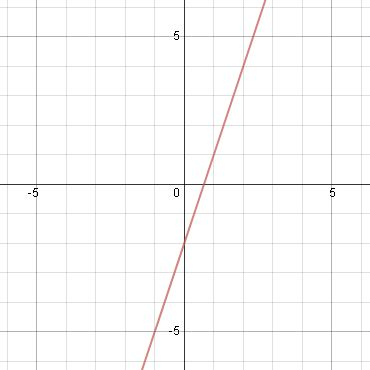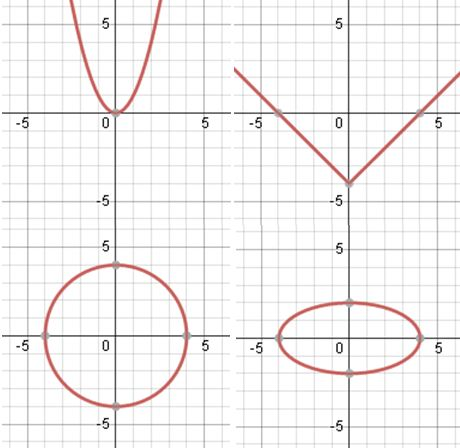# Linear and Nonlinear Functions

An error occurred trying to load this video.

Try refreshing the page, or contact customer support.

Coming up next: Graph Functions by Plotting Points

### You're on a roll. Keep up the good work!

Replay
Your next lesson will play in 10 seconds
• 0:00 Functions
• 1:00 Linear Functions
• 1:30 Nonlinear Functions
• 2:00 Equations
• 4:30 Lesson Summary
Save Save

Want to watch this again later?

Timeline
Autoplay
Autoplay
Speed Speed

#### Recommended Lessons and Courses for You

Lesson Transcript
Instructor: Yuanxin (Amy) Yang Alcocer

Amy has a master's degree in secondary education and has taught math at a public charter high school.

After watching this video lesson, you'll be able to tell the differences between linear and nonlinear functions. You'll also be able to identify both types just by looking at graphs.

## Functions

What is the one thing that you end up working with the most in math? Functions! In this lesson, you will learn the two types of functions that are out there in the world of math, including the differences between the two and what kinds of equations you can expect to see for both.

The two types are linear functions and nonlinear functions. Linear functions are the functions that graph out to a straight line. Nonlinear functions are the functions that don't graph out to a straight line. Why should you learn this? Because you'll be able to solve problems much more easily if you know what kind of function you're dealing with. This is so because you will have different formulas for each type of function. Some formulas are for working with linear functions only and some other formulas are for working with nonlinear functions only. So, being able to spot the type of function you are working with right away will help you solve your problems faster.

Let's get started.

## Linear Functions

As the name implies, linear functions are functions of lines; straight lines, to be exact. This graph here shows what a linear function looks like when graphed. All linear functions will graph out to a straight line. The location of the line may be different and the slant may be different, but the line will always be straight. Just tell yourself that if you can draw the function's line by making just one line with a ruler, then it is linear.## Nonlinear Functions

Nonlinear functions, on the other hand, graph out to anything but a straight line. Nonlinear functions can graph out as curves, two straight lines that meet somewhere, anything but a straight line is possible with nonlinear functions. All of these graphs here are examples of nonlinear functions.You can't draw any of these with just one line from a ruler. You either have to move your ruler or you have to use another tool to draw the curves.

## Equations

The equations for both types are also different. What you can expect to see in one, you can't expect to see in the other. Both linear and nonlinear functions use variables, typically x and y, but how the equations are written are very different.

Linear functions can come in any one of three forms. The first form is called the slope-intercept form. This form is written y = mx + b, where m is the slope, or slant of the line, and the b is the y-intercept, or where the line crosses the y-axis.

The second form is called the point-slope form. This form is y - y sub 1 = m(x - x sub 1), where y sub 1 and x sub 1 are the y and x coordinates of a point on the line and the m is the slope.

The third form is called the general form. It is written as Ax + By + C = 0, where A, B, and C are numbers, and with A and B never both being 0. If A is 0, then B cannot be 0 and vice versa.

To unlock this lesson you must be a Study.com Member.

### Register to view this lesson

Are you a student or a teacher?

#### See for yourself why 30 million people use Study.com

##### Become a Study.com member and start learning now.
Back
What teachers are saying about Study.com

### Earning College Credit

Did you know… We have over 200 college courses that prepare you to earn credit by exam that is accepted by over 1,500 colleges and universities. You can test out of the first two years of college and save thousands off your degree. Anyone can earn credit-by-exam regardless of age or education level.